# Diode Clipper Circuits: Design & Demonstration

Published  August 2, 2019   0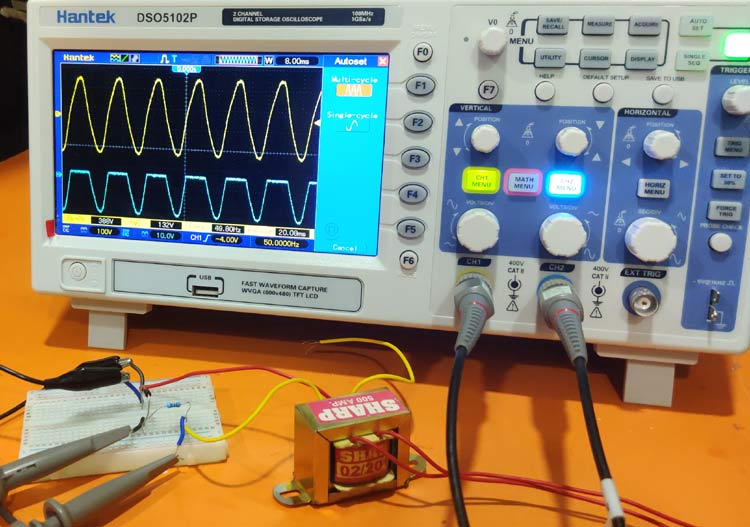As the name suggests, Clipper circuit is used to “clip” a portion of input signal without distorting the remaining part of the waveform. It is a wave shaping circuit. This can be very useful in the circuits where the input signal attains a higher value of voltage than the expected one. These circuits can be implemented in various ways according to the configuration or function of the diode.

The clipping is done either of positive or negative cycle by changing the configuration of the diode. So there are positive or negative clippers depending upon which cycle is being clipped. Here we will explain and demonstrate different types of clippers by using an Oscilloscope.

The main types of clippers are:

1. Series Positive Clipper
2. Series Positive Clipper with Bias Voltage
3. Series Negative Clipper
4. Series Negative Clipper with Bias Voltage
5. Shunt Positive Clipper
6. Shunt Positive Clipper with Bias Voltage
7. Shunt Negative Clipper
8. Shunt Negative Clipper with Bias Voltage
9. Combinational Clipper

### 1. Series Positive Clipper

As the name suggests, this circuit clips off the positive half cycle of the input signal. The diode is connected in series with output as shown in the figure below: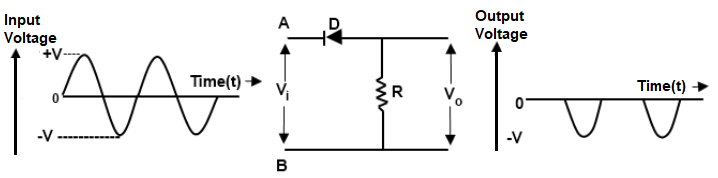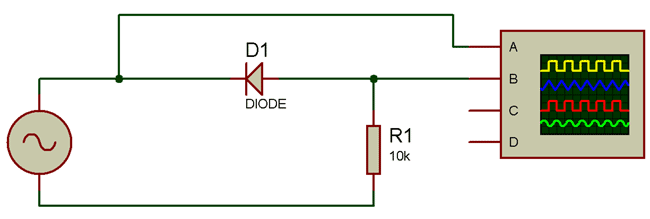To design the circuit, just follow the circuit diagram above. First connect the 12V terminal of transformer to diode’s negative end and connect a 10K resistor to diode’s positive end and then connect the 0V terminal of transformer to the other end of resistor. Now connect the first channel of oscilloscope to input side and the second channel to the output side. Turn on the transformer and oscilloscope. And, you’ll see the output signal’s positive half cycle clipped off.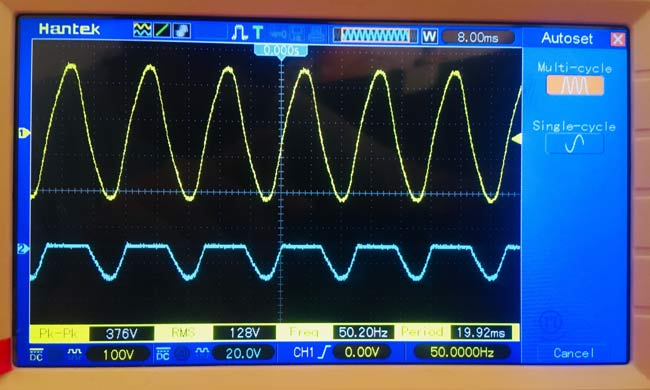During the positive half cycle the diode is in reverse bias so there is no output voltage, and during the negative half cycle the diode goes in forward bias and the voltage drop occurs across output. Hence we see the positive half cycle clipped off.

### 2. Series Positive Clipper with Bias Voltage

Many times we only need just a small portion of the signal to be clipped off. To do that bias voltage is used. So when we connect the bias voltage with the resistance then the output voltage is the difference between input voltage and bias voltage. This is how the positive half clips off to the desired voltage level. If you provide negative voltage (Figure 2), it will clip some part of negative cycle as shown below because the negative voltage will add up with the input voltage.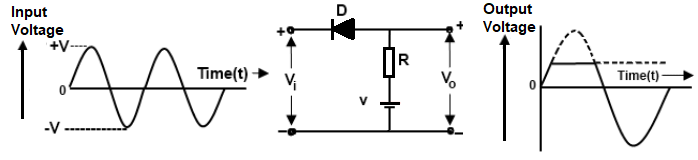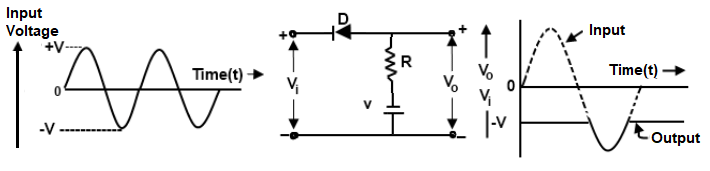### 3. Series Negative Clipper

As the name suggests, this circuit clips off the negative half cycle of the input signal. The diode is connected in series with output as shown in the figure below: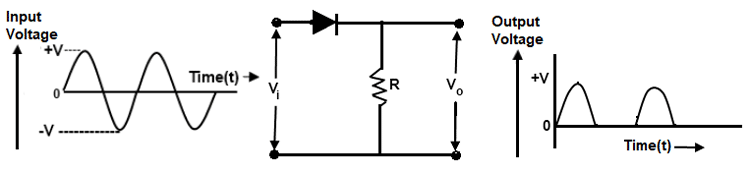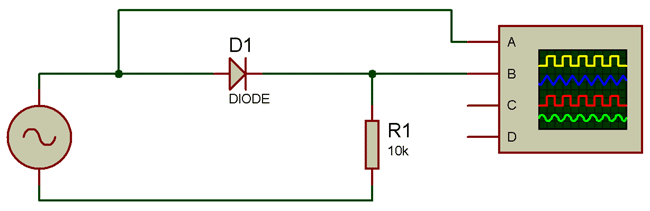To design the circuit, just follow the circuit diagram above. Firstly connect the 12V terminal of transformer to diode’s positive end and connect a 10K resistor to diode’s negative end and then connect the 0V terminal of transformer to the other end of resistor. Now connect the first channel of oscilloscope to input side and the second channel to the output side. Turn on the transformer and oscilloscope. And, you’ll see the output signal’s negative half cycle clipped off.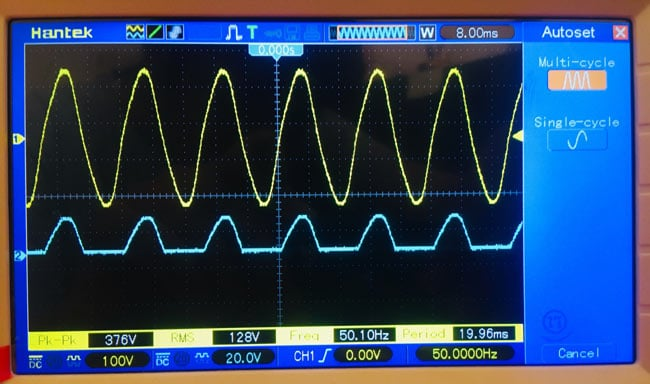During the positive half cycle the diode is in forward bias so, the voltage drop occurs across output and during the negative half cycle the diode goes in reverse bias and there is no output voltage across output. Hence we see the negative half cycle clipped off.

### 4. Series Negative Clipper with Bias Voltage

This works on the same principle as the series positive biased clipper. But here negative bias voltage is used to clip of the negative portion of the signal because the positive bias voltage will get added to the input voltage.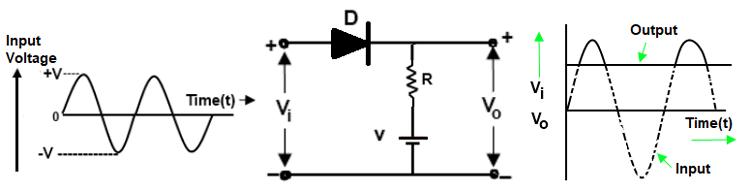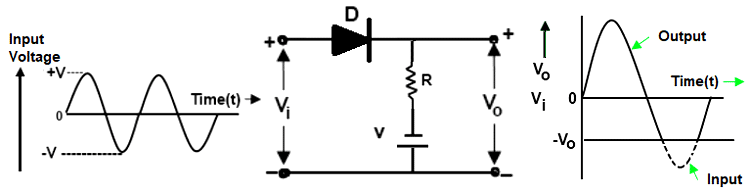### 5. Shunt Positive Clipper

In Shunt/Parallel Clipper, the diode is connected to the output side and the resistance is connected at input side. It is called parallel because the output is developed in parallel to the diode. The circuit diagram is shown below: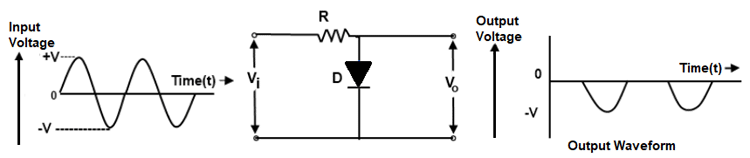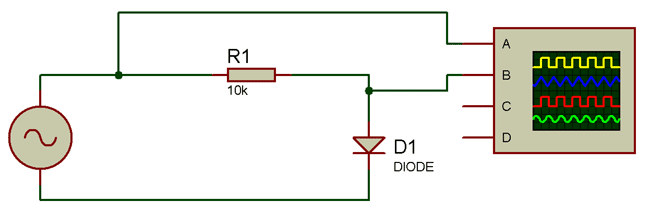To design the circuit, follow the circuit diagram shown above. Firstly connect the 12V terminal of transformer to 10K resistor and connect the diode’s positive end to this resistor and then connect the 0V terminal of transformer to the negative end of diode. Now connect the first channel of oscilloscope to input side and the second channel to the output side. Turn on the transformer and oscilloscope. And, you’ll see the output signal’s positive half cycle clipped off.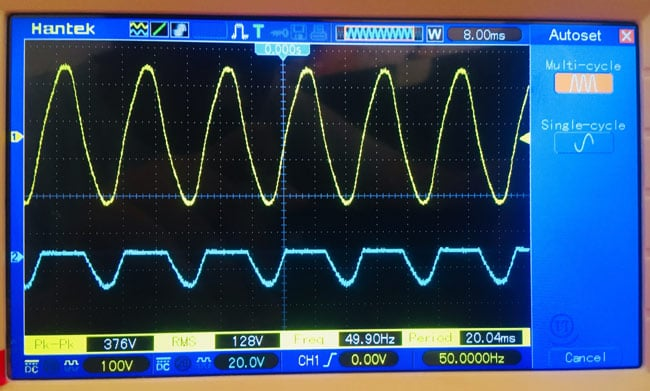During the positive half cycle the diode is in forward bias so, it acts as a short circuit and there is no output voltage in case of short circuit. Now during the negative half cycle the diode goes in reverse bias and acts as open circuit and the output voltage becomes equal to input voltage. Hence we see the positive half cycle clipped off.

### 6. Shunt Positive Clipper with Bias Voltage

This type of clipper also work same as the biased clippers discussed earlier but this time the bias voltage is connected with diode. So in positive biasing it clips off only the positive part but while it is biased negatively it also clips off some part of negative half cycle as shown in the diagram below.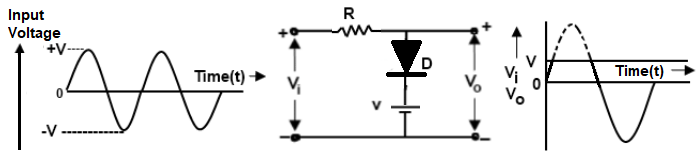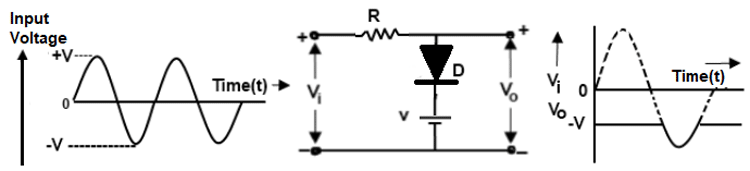### 7. Shunt Negative Clipper

This filter is designed same as the shunt positive clipper, just the diode is connected in reverse. The circuit diagram is given below: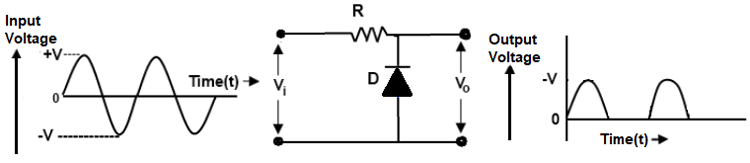First connect the 12V terminal of transformer to 10K resistor and connect the diode’s negative end to this resistor and then connect the 0V terminal of transformer to the positive end of diode. Now connect the first channel of oscilloscope to input side and the second channel to the output side. Turn on the transformer and oscilloscope. And, you’ll see the output signal’s negative half cycle clipped off.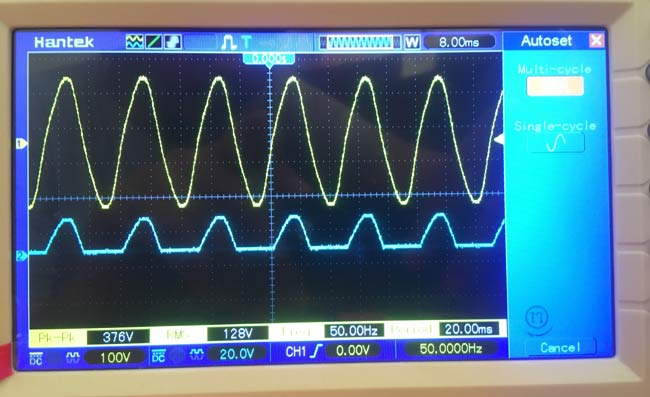During the positive half cycle the diode is in reverse bias so, it acts as an open circuit and the output voltage becomes equal to input voltage. Now during the negative half cycle the diode goes in forward bias and acts as short circuit and there is no output voltage in case of short circuit. Hence we see the negative half cycle clipped off.

### 8. Shunt Negative Clipper with Bias Voltage

They are also similar to series negative biased clippers but this time the voltage is connected with diode. You must use the negative bias voltage to achieve the clipping in negative cycle and positive bias to clip positive cycle.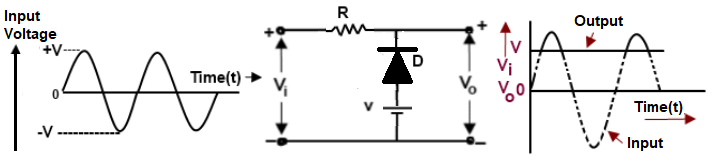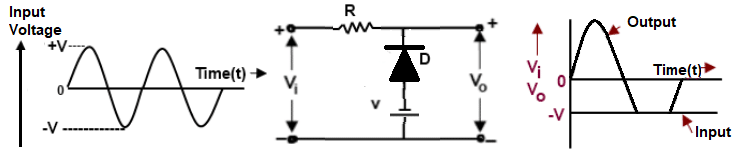### 9. Combinational Clippers

These clippers are used to clip off both the positive and negative cycles to some level. To accomplish this, two diodes are used in opposite directions. To regulate the clipping a bias voltage can be applied so that the clipping is done to the voltage difference between input and bias voltage. The circuit diagram is given below: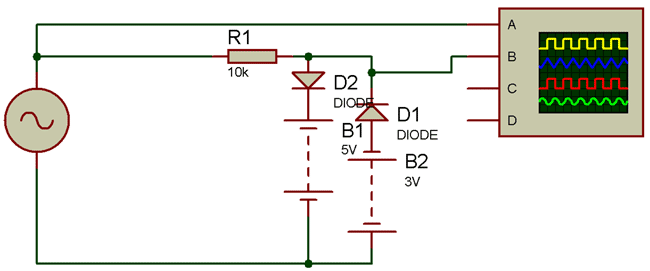Just follow the circuit diagram shown above. This circuit is same as the above parallel/shunt circuits but we’ve used two diodes here. We’ve made the circuit without using the bias voltage, so in output both the cycles will be clipped off.

During positive half cycle the D2 is forward biased and D1 is in reverse bias. So D2 will become short circuit and D1 will be open circuit. And similarly for negative half cycle the opposite of the above condition will occur. But the output will be at the voltage difference level and as we’ve not used the bias voltage so both the cycles will get clipped off.